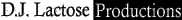Who is on now? Guests: 0 Members:HomepageAccount

My Account
Inbox
Compose
New UserTabs Listing

List All Tabs
Tabs by Letter
View Files
Search Tabs
Random TabContribute

Forum
My Drafts
Post a FileResources

Site Blog
Tab Guide
Player List
Member List
Member Map
Tab Rulers
Mobile Site
Tab ToolWebsite

StoreAdd to Favorites | Post File | Print | Send to a Friend | Report Tab

 Tweet Song Name: Breakfast Theme (PeeWee's Big Adventure) By: Danny Elfman Posted By: mmolino54 Difficulty: Any Key: C Genre: Theme Harp Type: Chromatic Audio: Breakfast Theme (PeeWee's Big Adventure) Created: 2008-01-08 04:01:54 Modified: 0000-00-00 00:00:00 Rating: Login to Vote Avg Rating: More Votes Needed Fav Count: 0
 Ad: Want to make Breakfast Theme (PeeWee's Big Adventure) sound awesome on your harmonica? Master tight single notes, rock solid bending and more with easy video lessons ->click here.

 Song: This song made a resurgence in Dec. '07 thanks to Visa using it in oneof their holiday advertisements on TV. PART A (play 2x) <-7 <-7 <-7 <-7 <-7 -7 <-7 -7 <+7 <+7 <+7 <+7 <+7 +7 <+7 -7 <-7 <-7 <-7 <-7 <-7 -7 <-7 -7 <+7 PART B <-3 <-3 <-3 <-3 <-3 -3 <+3 <-2 <-3 <-3 -3 <+3 <-2 <-3 -3 <+3 <-2 <-1 <-1 <-1 <-1 <-3 <-3 <-3 <-3 <-3 -3 <+3 <-2 <-3 <-3 -3 <+3 <-2 <-3 -3 <+3 <-2 PART C <-5 -5 <+5 <-3 -3 <-3 <+5 -5 <-5 -5 <+5 <-3 -3 <-3 <+5 -5 <-5 -5 <+5 <-3 -3     PART D <-3 <-3 <-3 <-3 <-3 -3 <+3 <-2 <-3 <-3 -3 <+3 <-2 <-3 -3 <+3 <-2 <-1 <-1 <-1 <-1 <-3 <-3 <-3 <-3 <-3 -3 <+3 <-2 <-3 <-3 -3 <+3 <-2 <-3 -3 <+3 <-2 <-1 <-1 <-1 <-1 <-3 <-3 <-3 -3 <+3 <-2 PART C (same as above) <-5 -5 <+5 <-3 -3 <-3 <+5 -5 <-5 -5 <+5 <-3 -3 <-3 <+5 -5 <-5 -5 <+5 <-3 -3   PART E <-7 <-7 <-7 <-7 <-7 -7 <-7 -7 <+7 <+7 <+7 <+7 <+7 +7 <+7 -7 <-7 <-7 <-7 <-7 <-7 -7 <-7 -7 <+7 <-7 <-7 <-7 <-7 <-7 -7 <-7 -7 <+7 <+7 <+7 <+7 <+7 +7 <+7 -7 <-7 PART B (same as above) <-3 <-3 <-3 <-3 <-3 -3 <+3 <-2 <-3 <-3 -3 <+3 <-2 <-3 -3 <+3 <-2 <-1 <-1 <-1 <-1 <-3 <-3 <-3 <-3 <-3 -3 <+3 <-2 <-3 <-3 -3 <+3 <-2 <-3 -3 <+3 <-2 PART C (same as above) <-5 -5 <+5 <-3 -3 <-3 <+5 -5 <-5 -5 <+5 <-3 -3 <-3 <+5 -5 <-5 -5 <+5 <-3 -3   PART F <-3 <-3 <-3 <-3 <-3 -3 <+3 <-2 <-3 <-3 -3 <+3 <-2 <-3 -3 <+3 <-2 <-1 <-1 <-1 <-1 <-3 <-3 <-3 <-3 <-3 -3 <+3 <-2 <-3 PART G <-7 <-7 <-7 <-7 <-7 -7 <-7 -7 <+7 <+7 <+7 <+7 <+7 +7 <+7 -7 <-7 <-7 <-7 <-7 <-7 -7 <-7 -7 <+7 <-7 <-7 <-7 <-7 <-7 -7 <-7 -7 <+7 <+7 <+7 <+7 <+7 +7 <+7 -7  <-7 <-7 <-7 <-7 <-7 -7 <-7 -7 <-7 -7 <-7 -7 <-7